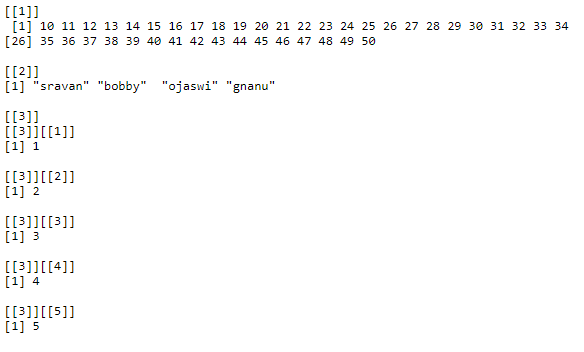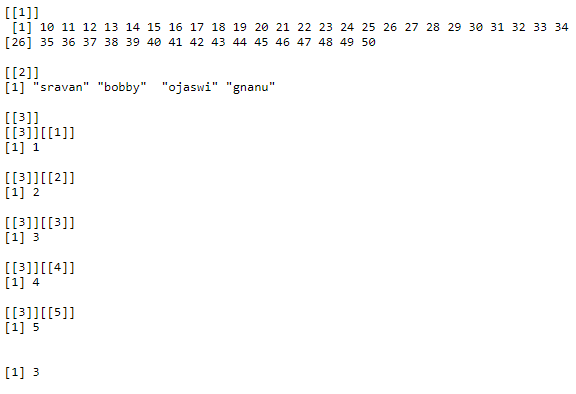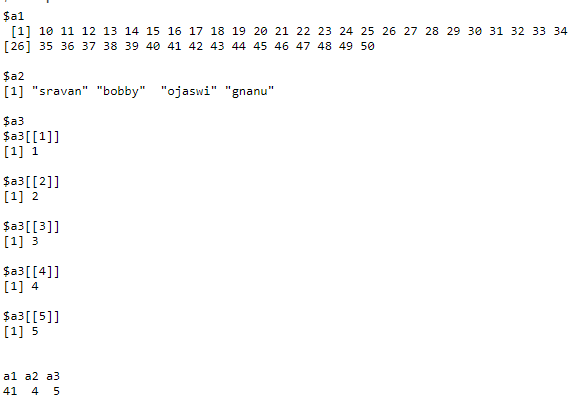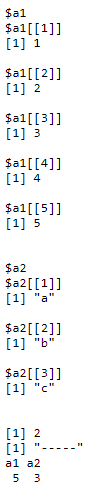Open In App

# Count Number of List Elements in R

In this article, we are going to count the elements in a list and elements in a nested list in R Programming Language. So we are going to use length() and lengths() to find the elements count in a list.

Steps –

• Create a list with vectors/list/range operator
• Find the count of elements using the length and lengths function.

Syntax: list(value1,value2,…,value)

values can be range operator or vector.

Let’s create a list using the range, vector, and list.

## R

 `# range from 10 to 50``values = 10:50`` ` `# vector elements of character type``names = ``c``(``"sravan"``, ``"bobby"``, ``"ojaswi"``, ``"gnanu"``)`` ` `# data1 with list of elements``data1 = ``list``(1, 2, 3, 4, 5)`` ` `# give input to the data which is a list``data = ``list``(values, names, data1)`` ` `# display``print``(data)`

Output:Example 1: Using length() function.

Length function is used to count the elements in the list

Syntax: length(listname)

return value: integer

Below is the implementation:

## R

 `# range from 10 to 50``values = 10:50`` ` `# vector elements of character type``names = ``c``(``"sravan"``, ``"bobby"``, ``"ojaswi"``, ``"gnanu"``)`` ` `# data1 with list of elements``data1 = ``list``(1, 2, 3, 4, 5)`` ` `# give input to the data which is a list``data = ``list``(values, names, data1)`` ` `# display``print``(data)`` ` `# count elements using length function``print``(``length``(data))`

Output:Example 2: For finding the length of each data in a list (nested list) we will use lengths() function

Syntax: lengths(list_name)

Below is the implementation:

## R

 `# range from 10 to 50``values = 10:50`` ` `# vector elements of character type``names = ``c``(``"sravan"``, ``"bobby"``, ``"ojaswi"``, ``"gnanu"``)`` ` `# data1 with list of elements``data1 = ``list``(1, 2, 3, 4, 5)`` ` `# give input to the data which is a list``data = ``list``(a1 = values, a2 = names, a3 = data1)`` ` `# display``print``(data)`` ` `# count elements in each nested  using lengths function``print``(``lengths``(data))`

Output:Example 3: R program count elements in a nested list

## R

 `# data1 with list of elements``data1 = ``list``(1, 2, 3, 4, 5)`` ` `# data2 with list of elements``data2 = ``list``(``"a"``, ``'b'``, ``'c'``)`` ` `# give input to the data which is a list``data = ``list``(a1 = data1, a2 = data2)`` ` `# display``print``(data)`` ` `# count elements in each nested  using length function``print``(``length``(data))``print``(``"-----"``)`` ` `# count elements in each nested  using lengths function``print``(``lengths``(data))`

Output: